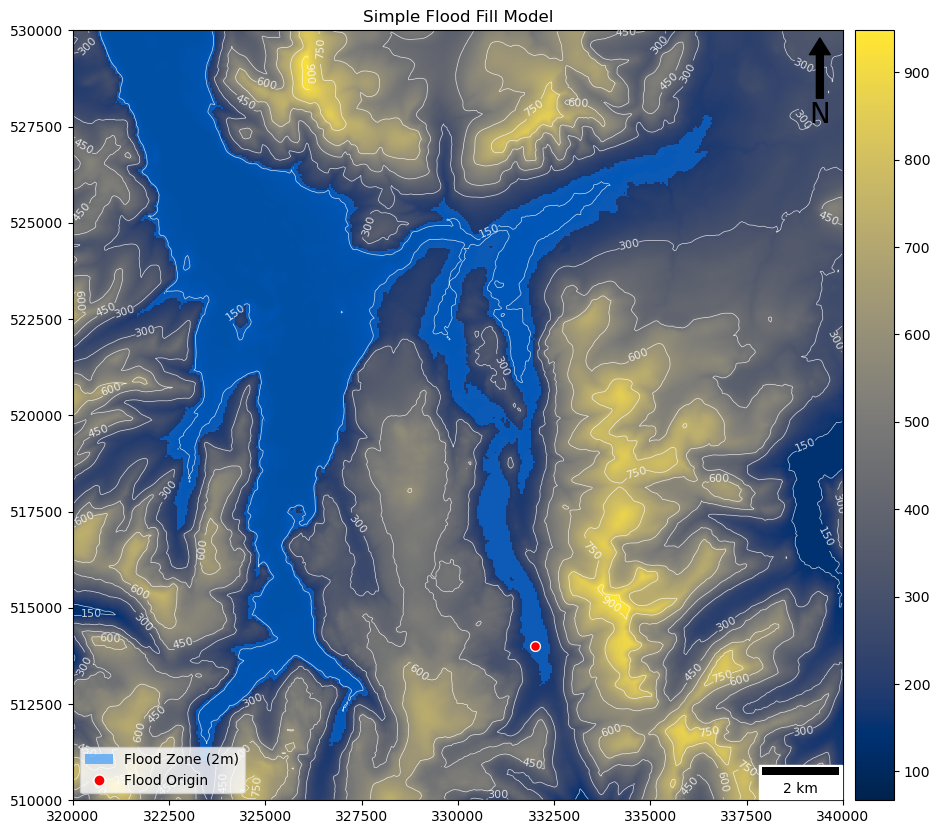# Practical 6 Code Solution``````"""
Understanding GIS: Practical 6
@author jonnyhuck

Simple implementation of a flood fill model

References:
https://docs.python.org/3/tutorial/errors.html
https://matplotlib.org/stable/gallery/color/colormap_reference.html
https://matplotlib.org/api/_as_gen/matplotlib.axes.Axes.contour.html#matplotlib.axes.Axes.contour

Note:
You *cannot* use a try-except statement to catch an Index Error, as negative numbers are valid
and so just wrap around to the other side of the dataset

New Topics:
Rasters
Sets
numpy arrays
with statements
continue statements
"""

from numpy import zeros
from math import floor, ceil
from geopandas import GeoSeries
from shapely.geometry import Point
from matplotlib.lines import Line2D
from matplotlib.patches import Patch
from rasterio import open as rio_open
from matplotlib.colors import Normalize
from matplotlib.cm import ScalarMappable
from rasterio.plot import show as rio_show
from matplotlib.pyplot import subplots, savefig
from matplotlib_scalebar.scalebar import ScaleBar
from matplotlib.colors import LinearSegmentedColormap

def flood_fill(depth, x0, y0, d, dem_data):
"""
Calculate flooding extent from a point location
"""

# create output array at the same dimensions as the d
flood_layer = zeros(dem_data.shape)

# convert from coordinate space to image space
r0, c0 = d.index(x0, y0)

# set for cells already assessed
assessed = set()

# set for cells to be assessed
to_assess = set()

# calculate the maximum elevation of the flood
flood_extent = dem_data[(r0, c0)] + depth

# keep looping as long as there are cells left to be checked
while to_assess:

# get the next cell to be assessed (removing it from the to_assess set)
r, c = to_assess.pop()

# is the elevation low enough for this cell to be flooded?
if dem_data[(r, c)] <= flood_extent:

# mark the current cell as flooded
flood_layer[(r, c)] = 1

# loop through all neighbouring cells
for r_adj, c_adj in [(-1, -1), (-1, 0), (-1, 1), (0, -1), (0, 1), (1, -1), (1, 0), (1, 1)]:

# get current neighbour

# make sure that the location is wihin the bounds of the dataset and has not been assessed previously...
if 0 <= neighbour < dem.height and 0 <= neighbour < dem.width and neighbour not in assessed:

# ...then add to the set for assessment

# when complete, return the result
return flood_layer

# set the depth of the flood
FLOOD_DEPTH = 2

# set origin for the flood as a tuple
LOCATION = (332000, 514000)

# open the raster dataset
# with rio_open('../data/helvellyn/Helvellyn.tif') as dem:   # 5m  resolution
with rio_open("../data/helvellyn/Helvellyn-50.tif") as dem:  # 50m resolution

# read the data out of band 1 in the dataset

# calculate the flood
output = flood_fill(FLOOD_DEPTH, LOCATION, LOCATION, dem, dem_data)

# output image
fig, my_ax = subplots(1, 1, figsize=(16, 10))
my_ax.set_title("Simple Flood Fill Model")

# draw dem
rio_show(
dem_data,
ax=my_ax,
transform = dem.transform,
cmap = 'cividis',
)

# draw dem as contours
rio_show(
dem_data,
ax=my_ax,
contour=True,
transform = dem.transform,
colors = ['white'],
linewidths = [0.5],
)

rio_show(
output,
ax=my_ax,
transform=dem.transform,
cmap = LinearSegmentedColormap.from_list('binary', [(0, 0, 0, 0), (0, 0.5, 1, 0.5)], N=2)
)

GeoSeries(Point(LOCATION)).plot(
ax = my_ax,
markersize = 50,
color = 'red',
edgecolor = 'white'
)

x, y, arrow_length = 0.97, 0.99, 0.1
my_ax.annotate('N', xy=(x, y), xytext=(x, y-arrow_length),
ha='center', va='center', fontsize=20, xycoords=my_ax.transAxes)